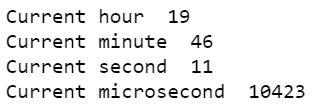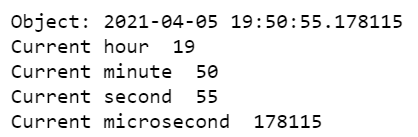# Python program to print current hour, minute, second and microsecond

• Last Updated : 09 Dec, 2021

In this article, we are going to discuss how to print current hour, minute, second, and microsecond using Python. In order to print hour, minute and microseconds we need to use DateTime module in Python.

### Methods used

• datetime.now().hour(): This method returns the current hour value of the datetime object.
• datetime.now().minute(): This method returns the current minute value of the datetime object.
• datetime.now().second(): This method returns the current second value of the datetime object.
• datetime.now().microsecond(): This method returns the current microsecond value of the datetime object.

Below are the various implementations using examples that depict how to print current hour, minute, second, and microsecond in python.

Example 1: To print time, hour, minute, second, and microsecond

## Python3

 `# importing datetime module``from` `datetime ``import` `datetime ` `# now is a method in datetime module is``# used to retrieve current data,time``myobj ``=` `datetime.now()` `# printing current hour using hour``# class``print``(``"Current hour "``, myobj.hour) ` `# printing current minute using minute``# class``print``(``"Current minute "``, myobj.minute)` `# printing current second using second``# class``print``(``"Current second "``, myobj.second)` `# printing current microsecond using``# microsecond class``print``(``"Current microsecond "``, myobj.microsecond)`

Output:Example 2: To print object, time, hour, minute, second, and microsecond.

## Python3

 `# importing datetime module``from` `datetime ``import` `datetime ` `# now is a method in datetime module is``# used to retrieve current data,time``myobj ``=` `datetime.now()`  `# printing the object itself``print``(``"Object:"``, myobj)` `# printing current hour using hour``# class``print``(``"Current hour "``, myobj.hour) ` `# printing current minute using minute``# class``print``(``"Current minute "``, myobj.minute)` `# printing current second using second``# class``print``(``"Current second "``, myobj.second)` `# printing current microsecond using microsecond``# class``print``(``"Current microsecond "``, myobj.microsecond)`

Output:My Personal Notes arrow_drop_up